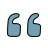### Evaluation of Number Sense on the Subject of Decimal Numbers of the Secondary Stage Students in Turkey

###### Author:

Number of pages:
Year-Number: 2012-Volume 4, Issue 2

### Abstract

The aim of this study is to examine number sense on the subject of decimal numbers of the secondary stage students in Turkey. Number sense, which can be described as well-understanding of numbers and calculations, is one of the main foci of mathematical education. When students improve their number sense, which are ability to estimate, mental computations and analytical thinking that will increase their use of mathematics in daily life. So as to measure students’ number senses about decimal numbers, a test called Number Sense Test about Decimal Numbers which was prepared by the researchers was applied to 573 students (Secondary-stage students: 6th, 7th and 8th grades.) in 6 schools from the different regions in Turkey. Later on, in order to find out the possible solutions, 9 students were interviewed. As a result of the study, students’ number senses on decimal numbers turn out to be very low. Root causes of these can be considered as the use of rule-based solution techniques, imperfect knowledge and misinformation about decimal numbers. This type of sense has a good correlation with students’ ages, however, it turns out that there is no correlation between genders of the students and their number senses. In addition to these, medium level correlation was found out between mathematical achievement and number sense on decimal numbers. It is believed that after the importance of number sense is acknowledged, the problems of failure will be prevented.

### Abstract

The aim of this study is to examine number sense on the subject of decimal numbers of the secondary stage students in Turkey. Number sense, which can be described as well-understanding of numbers and calculations, is one of the main foci of mathematical education. When students improve their number sense, which are ability to estimate, mental computations and analytical thinking that will increase their use of mathematics in daily life. So as to measure students’ number senses about decimal numbers, a test called Number Sense Test about Decimal Numbers which was prepared by the researchers was applied to 573 students (Secondary-stage students: 6th, 7th and 8th grades.) in 6 schools from the different regions in Turkey. Later on, in order to find out the possible solutions, 9 students were interviewed. As a result of the study, students’ number senses on decimal numbers turn out to be very low. Root causes of these can be considered as the use of rule-based solution techniques, imperfect knowledge and misinformation about decimal numbers. This type of sense has a good correlation with students’ ages, however, it turns out that there is no correlation between genders of the students and their number senses. In addition to these, medium level correlation was found out between mathematical achievement and number sense on decimal numbers. It is believed that after the importance of number sense is acknowledged, the problems of failure will be prevented.

### Keywords

• Article Statistics
•Quote